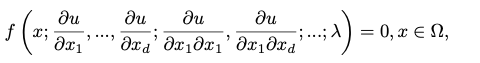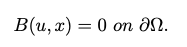# PhysicsInformedNN Discretizer for PDESystems

Using the PINNs solver, we can solve general nonlinear PDEs:with suitable boundary conditions:where time t is a special component of x, and Ω contains the temporal domain.

PDEs are defined using the ModelingToolkit.jl PDESystem:

@named pde_system = PDESystem(eq,bcs,domains,param,var)

Here, eq is the equation, bcs represents the boundary conditions, param is the parameter of the equation (like [x,y]), and var represents variables (like [u]). For more information, see the ModelingToolkit.jl PDESystem documentation.

## The PhysicsInformedNN Discretizer

NeuralPDE.PhysicsInformedNNType

julia PhysicsInformedNN(chain, strategy; initparams = nothing, phi = nothing, paramestim = false, additionalloss = nothing, adaptiveloss = nothing, logger = nothing, log_options = LogOptions(), iteration = nothing, kwargs...) where {iip}

A discretize algorithm for the ModelingToolkit PDESystem interface which transforms a PDESystem into an OptimizationProblem using the Physics-Informed Neural Networks (PINN) methodology.

Positional Arguments

• chain: a vector of Flux.jl or Lux.jl chains with a d-dimensional input and a 1-dimensional output corresponding to each of the dependent variables. Note that this specification respects the order of the dependent variables as specified in the PDESystem.
• strategy: determines which training strategy will be used. See the Training Strategy documentation for more details.

Keyword Arguments

• init_params: the initial parameters of the neural networks. This should match the specification of the chosen chain library. For example, if a Flux.chain is used, then init_params should match Flux.destructure(chain) in shape. If init_params is not given, then the neural network default parameters are used. Note that for Lux, the default will convert to Float64.
• phi: a trial solution, specified as phi(x,p) where x is the coordinates vector for the dependent variable and p are the weights of the phi function (generally the weights of the neural network defining phi). By default this is generated from the chain. This should only be used to more directly impose functional information in the training problem, for example imposing the boundary condition by the test function formulation.
• adaptive_loss: the choice for the adaptive loss function. See the adaptive loss page for more details. Defaults to no adaptivity.
• additional_loss: a function additional_loss(phi, θ, p_) where phi are the neural network trial solutions, θ are the weights of the neural network(s), and p_ are the hyperparameters of the OptimizationProblem. If param_estim = true, then θ additionally contains the parameters of the differential equation appended to the end of the vector.
• param_estim: whether the parameters of the differential equation should be included in the values sent to the additional_loss function. Defaults to true.
• logger: ?? needs docs
• log_options: ?? why is this separate from the logger?
• iteration: used to control the iteration counter???
• kwargs: Extra keyword arguments which are splatted to the OptimizationProblem on solve.
NeuralPDE.PhiType

An encoding of the test function phi that is used for calculating the PDE value at domain points x

Fields:

• f: A representation of the chain function. If FastChain, then f(x,p), if Chain then f(p)(x) (from Flux.destructure)
• st: The state of the Lux.AbstractExplicitLayer. If a Flux.Chain then this is nothing. It should be updated on each call.
SciMLBase.discretizeMethod
prob = discretize(pde_system::PDESystem, discretization::PhysicsInformedNN)

Transforms a symbolic description of a ModelingToolkit-defined PDESystem and generates an OptimizationProblem for Optimization.jl whose solution is the solution to the PDE.

## symbolic_discretize and the lower-level interface

SciMLBase.symbolic_discretizeMethod
prob = symbolic_discretize(pde_system::PDESystem, discretization::PhysicsInformedNN)

symbolic_discretize is the lower level interface to discretize for inspecting internals. It transforms a symbolic description of a ModelingToolkit-defined PDESystem into a PINNRepresentation which holds the pieces required to build an OptimizationProblem for Optimization.jl whose solution is the solution to the PDE.

For more information, see discretize and PINNRepresentation.

NeuralPDE.PINNRepresentationType

PINNRepresentation

An internal reprsentation of a physics-informed neural network (PINN). This is the struct used internally and returned for introspection by symbolic_discretize.

Fields

• eqs

The equations of the PDE

• bcs

The boundary condition equations

• domains

The domains for each of the independent variables

• eq_params

???

• defaults

???

• default_p

???

• param_estim

Whether parameters are to be appended to the additional_loss

• additional_loss

The additional_loss function as provided by the user

• adaloss

• depvars

The dependent variables of the system

• indvars

The independent variables of the system

• dict_indvars

A dictionary form of the independent variables. Define the structure ???

• dict_depvars

A dictionary form of the dependent variables. Define the structure ???

• dict_depvar_input

???

• logger

The logger as provided by the user

• multioutput

Whether there are multiple outputs, i.e. a system of PDEs

• iteration

The iteration counter used inside of the cost function

• init_params

The initial parameters as provided by the user. If the PDE is a system of PDEs, this will be an array of arrays. If Lux.jl is used, then this is an array of ComponentArrays.

• flat_init_params

The initial parameters as a flattened array. This is the array that is used in the construction of the OptimizationProblem. If a Lux.jl neural network is used, then this flattened form is a ComponentArray. If the equation is a system of equations, then flat_init_params.depvar.x are the parameters for the neural network corresponding to the dependent variable x, and i.e. if depvar[i] == :x then for phi[i]. If param_estim = true, then flat_init_params.p are the parameters and flat_init_params.depvar.x are the neural network parameters, so flat_init_params.depvar.x would be the parameters of the neural network for the dependent variable x if it's a system. If a Flux.jl neural network is used, this is simply an AbstractArray to be indexed and the sizes from the chains must be remembered/stored/used.

• phi

The representation of the test function of the PDE solution

• derivative

The function used for computing the derivative

• strategy

The training strategy as provided by the user

• pde_indvars

???

• bc_indvars

???

• pde_integration_vars

???

• bc_integration_vars

???

• integral

???

• symbolic_pde_loss_functions

The PDE loss functions as represented in Julia AST

• symbolic_bc_loss_functions

The boundary condition loss functions as represented in Julia AST

• loss_functions

The PINNLossFunctions, i.e. the generated loss functions

NeuralPDE.PINNLossFunctionsType

PINNLossFunctions

The generated functions from the PINNRepresentation

Fields

• bc_loss_functions

The boundary condition loss functions

• pde_loss_functions

The PDE loss functions

• full_loss_function

The full loss function, combining the PDE and boundary condition loss functions. This is the loss function that is used by the optimizer.

• additional_loss_function

The wrapped additional_loss, as pieced together for the optimizer.

• datafree_pde_loss_functions

The pre-data version of the PDE loss function

• datafree_bc_loss_functions`

The pre-data version of the BC loss function